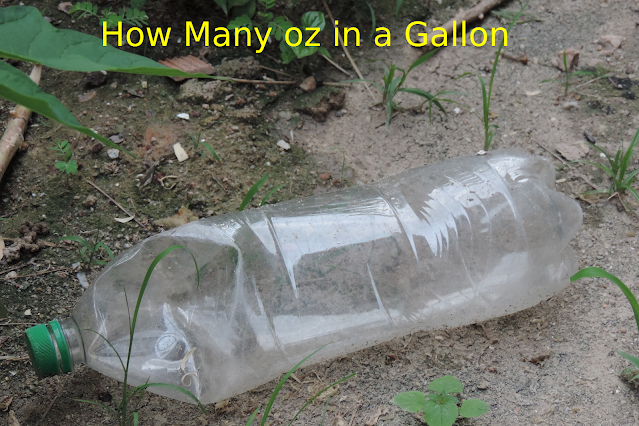# How Many oz in a Gallon

## How Many oz in a Gallon

If you're someone who cooks, bakes, or mixes drinks, then you know that precise measurements are crucial in achieving a perfect result. One of the most common measurements is the ounce and gallon. Knowing how many ounces are in a gallon can make a significant difference in your cooking or mixing process. In this article, we will explore everything you need to know about how many ounces are in a gallon.How Many oz in a Gallon?

Cooking is a science, and measurements are the key to success. In most cases, recipes call for specific measurements of ingredients, including ounces and gallons. In the culinary world, it's important to know how to convert measurements to achieve a precise result. This guide will provide a thorough understanding of how many ounces are in a gallon, including frequently asked questions.

## What is a Gallon?

Before we delve into how many ounces are in a gallon, it's essential to understand what a gallon is. A gallon is a unit of measurement used to quantify volume. It's commonly used in the United States and the United Kingdom to measure liquids such as water, milk, gasoline, and more. However, it's worth noting that the US and the UK use different types of gallons.

### Different Types of Gallons

There are two types of gallons: the US gallon and the imperial gallon. The US gallon is used in the United States and is equivalent to 128 fluid ounces. On the other hand, the imperial gallon is used in the United Kingdom and is equivalent to 160 fluid ounces.

## How Many oz in a Gallon?

Now that we understand what a gallon is let's explore how many ounces are in a gallon. As previously mentioned, the US gallon is equivalent to 128 fluid ounces, while the imperial gallon is equivalent to 160 fluid ounces. To convert gallons to ounces, all you have to do is multiply the number of gallons by the conversion factor (128 for the US gallon and 160 for the imperial gallon).

Here's a handy chart for easy reference:

GallonsOunces (US)Ounces (Imperial)
1128160
2256320
3384480
4512640
5640800

As you can see from the chart, there are 128 fluid ounces in one US gallon and 160 fluid ounces in one imperial gallon.

## Why is Knowing How Many Ounces in a Gallon Important?

Understanding how many ounces are in a gallon is important for a variety of reasons. For example, if you are cooking or baking, you may need to convert ounces to gallons or vice versa. Knowing how to make these conversions accurately can make a big difference in the success of your recipe. Additionally, if you work in a field that requires measurements such as construction or engineering, knowing how to convert between ounces and gallons is essential.

## FAQs

### Q1. Can I use a measuring cup to measure gallons?

No, you cannot use a measuring cup to measure gallons. Measuring cups are designed to measure smaller quantities such as cups, tablespoons, and teaspoons. To measure gallons, you will need a measuring jug or a kitchen scale.

### Q2. Can I convert ounces to gallons?

Yes, you can convert ounces to gallons. To convert ounces to gallons, divide the number of ounces by the conversion factor (128 for US gallons and 160 for imperial gallons).

### Q3. How many ounces are in half a gallon?

There are 64 fluid ounces in half a US gallon, while there are 80 fluid ounces in half an imperial gallon.

In conclusion, there are 128 fluid ounces in a US gallon, which is equivalent to approximately 8.34 pounds. Understanding how to convert between ounces and gallons is important for a variety of fields and activities, including cooking, baking, construction, and engineering. We hope that this article has provided you with a comprehensive and detailed answer to the question, "How many ounces are in a gallon?"

No Comment